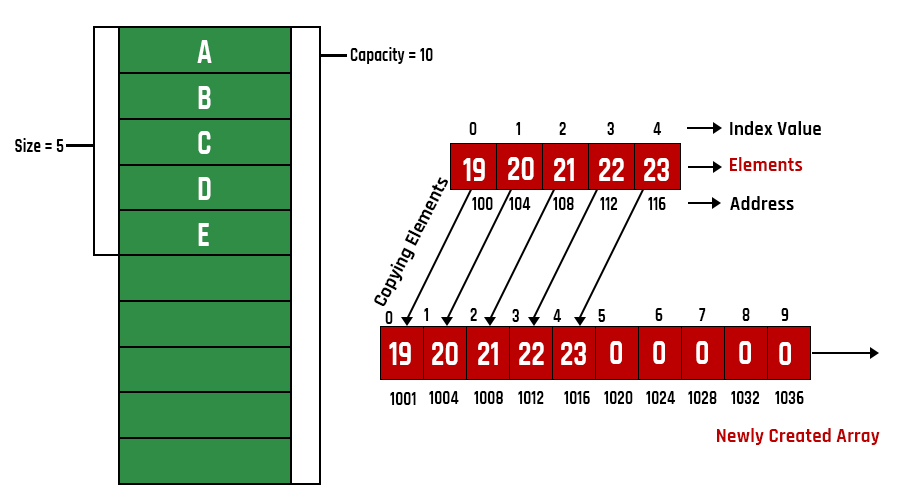# Creating a Dynamic Array in Java

• Difficulty Level : Easy
• Last Updated : 30 Jun, 2021

Arrays are linear data structures which means that similar types of elements will be inserted as known in a continuous manner. Now as we know there is an issue with arrays that size needs to be specified at the time of declaration or taken from the user in java which constricts ourselves. Hence, there arise dynamic arrays in java in which entries can be added as the array increases its size as it is full. The size of the new array increases to double the size of the original array. Now all elements are retained in a new array which is in specified array domain size and the rest are added after them in the newly formed array. This array keeps on growing dynamically.Procedure:

1. First, we declared an array of types int with the private access specifier.
2. Declare the count variable.
3. Create a constructor that initializes the array of the given length.
4. Here the magic comes with the method insert.
5. First, before we insert the value it checks for the length of the array and count variable if both are of the same size then the array is said to be full.
6. Then we create a new array whose size is twice the size of the previous array.
7. Just initialized the new array with the previous array and reinitialized it back to the previous array.

Implementation: Creating an Array class that declares the int arr and int count. We just created an array whenever the array is full the array will be resized.

Example

## Java

 `// Java Program to Create a Dynamic Array`   `// Class 1` `// Helper class` `class` `Array {`   `    ``// Member variables of this class` `    ``// Private access modifier` `    ``private` `int` `arr[];` `    ``private` `int` `count;`   `    ``// Note they can only be called through function`   `    ``// Method 1` `    ``// Inside helper class` `    ``// to compute length of an array` `    ``public` `Array(``int` `length) { arr = ``new` `int``[length]; }`   `    ``// Method 2` `    ``// Inside helper class` `    ``// To print array` `    ``public` `void` `printArray()` `    ``{`   `        ``// Iterating over array using for loop` `        ``for` `(``int` `i = ``0``; i < count; i++) {`   `            ``// Print the elements of an array` `            ``System.out.print(arr[i] + ``" "``);` `        ``}` `    ``}`   `    ``// Method 3` `    ``// Inside Helper class` `    ``public` `void` `insert(``int` `element)` `    ``{`   `        ``if` `(arr.length == count) {`   `            ``// Creating a new array double the size` `            ``// of array declared above` `            ``int` `newArr[] = ``new` `int``[``2` `* count];`   `            ``// Iterating over new array using for loop` `            ``for` `(``int` `i = ``0``; i < count; i++) {` `                ``newArr[i] = arr[i];` `            ``}`   `            ``// Assigning new array to original array` `            ``// created above` `            ``arr = newArr;` `        ``}`   `        ``arr[count++] = element;` `    ``}` `}`   `// Class 2` `// Main class` `public` `class` `GFG {`   `    ``// Main driver method` `    ``public` `static` `void` `main(String[] args)` `    ``{`   `        ``// Creating object of Array(user-defined) class` `        ``Array numbers = ``new` `Array(``3``);`   `        ``// Adding elements more than size specified above` `        ``// to the array to illustrate dynamic nature` `        ``// using the insert() method`   `        ``// Custom input elements` `        ``numbers.insert(``10``);` `        ``numbers.insert(``30``);` `        ``numbers.insert(``40``);` `        ``numbers.insert(``50``);`   `        ``// Calling the printArray() method to print` `        ``// new array been dynamically created` `        ``numbers.printArray();` `    ``}` `}`

Output

`10 30 40 50 `

My Personal Notes arrow_drop_up
Recommended Articles
Page :